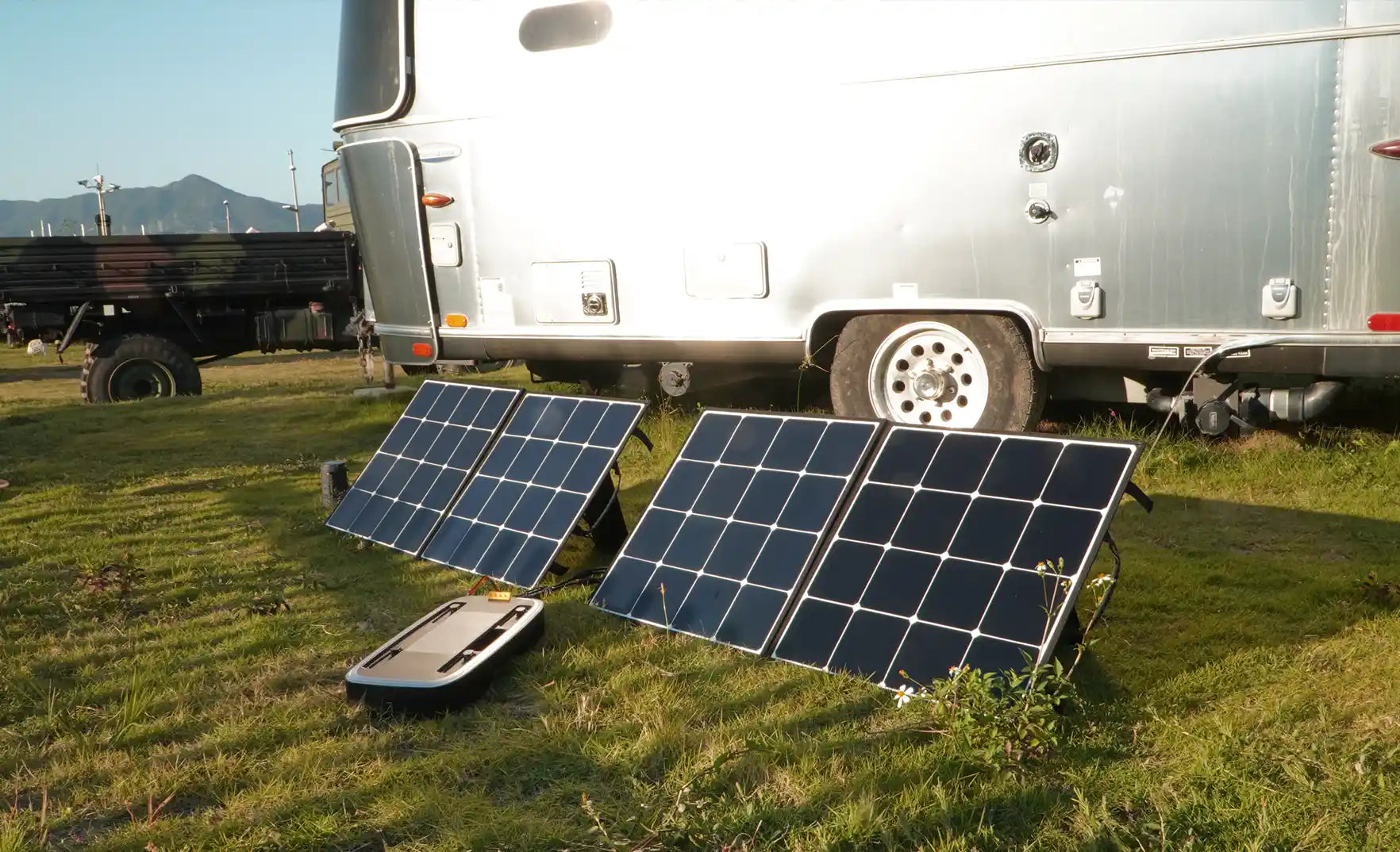# How do you install solar panels on your van?-STEP 3

### STEP 3: Decide how many panels you need.

There are so many different ways to install solar panels. We suggest you find a professional or expert friend to help you out. But first, we still hope you can understand how it works as much as possible.

We learned how to calculate the total energy you need for your van and what battery to store your solar energy in.

Solar panels are measured in watts. There are 100, 170, 200, 225, 265, 300, 335, and 360 watt solar panels. Then you can do the calculation. For example, if you need 300 WATT for your van, and each panel is 100 WATT, 300 divided by 100 equals 3, which means you need a 3 solar panel array.

Let's say you need 2600 WH/day.

Sunlight 10 hours. WATT equals WATTHOUR divided by HOUR (W = WH / HR).

2600/10 = 260W, which means you need 260W every hour. But this is just an average number that is not an accurate number.

Considering that the intensity of sunlight varies throughout the day, we count it as 60% energy. 433W = 260/60%. This means that we need at least 433W per day. Therefore, we suggest that you prepare 450W solar panels. Depending on your choice, you can have different combinations of solar panels. Just make sure the total power is more than the amount of energy you need.

Don't worry about overcharging the battery. When the battery is fully charged, it will stop absorbing solar energy. The solar panels will continue to generate electricity, but that electricity will not be used or stored.

To install a solar system, the first step is to calculate how much power you need in total. We can use the equation WATT-HOUR = WATT x HOUR / Day to get the number. Step 2 is to choose a power station that can store enough power to meet your needs. The power station stores should be more than what you need in total. Then you should be able to choose the solar panel. The total power it produces should also be more than what you really need.

Solar energy is clean energy, and it is one way to camp off-grid. While enjoying the beauty of nature, we should protect the environment. In general, we know the total power calculation, battery, and solar panel. We hope these will be helpful to your situation. Have wonderful trips.Advantages of the camper-van solar system

STEP 1: Calculate how much power you need for your van.

STEP 2: Choose a battery bank for your van.

STEP 3: Decide how many panels you need.